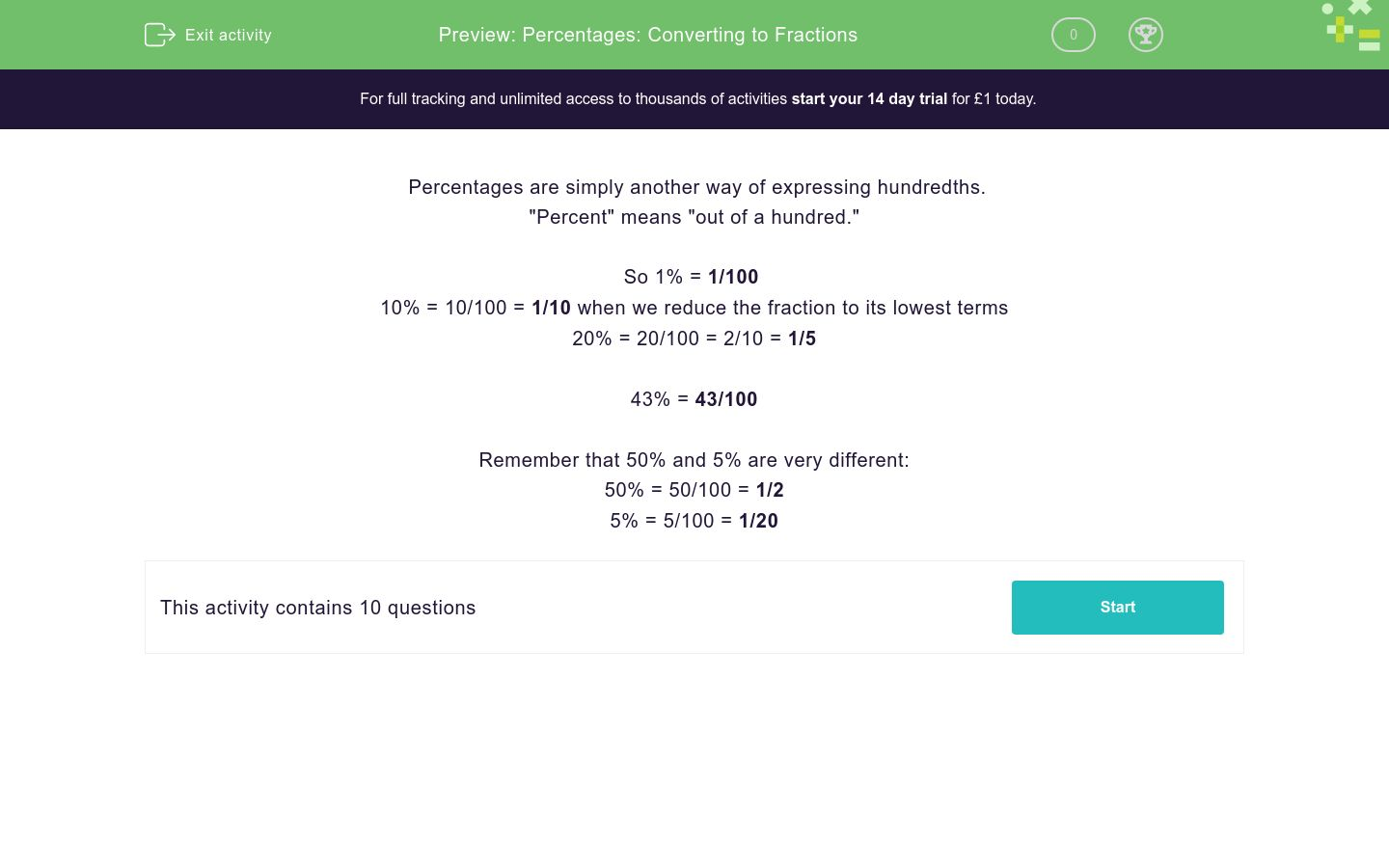# Percentages: Converting to Fractions

In this worksheet, students convert the given percentages into their fraction equivalents, giving answers in their lowest terms.Key stage:  KS 2

Curriculum topic:   Number: Fractions, Decimals and Percentages

Curriculum subtopic:   Recognise Percentages

Difficulty level:### QUESTION 1 of 10

Percentages are simply another way of expressing hundredths.

"Percent" means "out of a hundred."

So 1% = 1/100

10% = 10/100 = 1/10 when we reduce the fraction to its lowest terms

20% = 20/100 = 2/10 = 1/5

43% = 43/100

Remember that 50% and 5% are very different:

50% = 50/100 = 1/2

5% = 5/100 = 1/20

Convert the following percentage to a fraction in its lowest terms:

23%

1/23

23/100

23

Convert the following percentage to a fraction in its lowest terms:

20%

1/20

20/100

1/5

Convert the following percentage to a fraction in its lowest terms:

27%

1/27

27/100

1/3

Convert the following percentage to a fraction in its lowest terms:

25%

1/4

25/100

1/5

Convert the following percentage to a fraction in its lowest terms:

10%

1/10

10/100

1/5

Convert the following percentage to a fraction in its lowest terms:

50%

1/50

10/100

1/2

Convert the following percentage to a fraction in its lowest terms:

26%

13/50

26/100

1/26

Convert the following percentage to a fraction in its lowest terms:

44%

44/100

22/50

11/25

Convert the following percentage to a fraction in its lowest terms:

45%

9/50

45/100

9/20

Convert the following percentage to a fraction in its lowest terms:

75%

1/75

75/100

3/4

• Question 1

Convert the following percentage to a fraction in its lowest terms:

23%

23/100
EDDIE SAYS
23/100 is the fraction in lowest terms.
• Question 2

Convert the following percentage to a fraction in its lowest terms:

20%

1/5
EDDIE SAYS
20/100 = 1/5 20 divided by 20 = 1 100 divided by 20 = 5
• Question 3

Convert the following percentage to a fraction in its lowest terms:

27%

27/100
EDDIE SAYS
27/100 is the fraction in lowest terms.
• Question 4

Convert the following percentage to a fraction in its lowest terms:

25%

1/4
EDDIE SAYS
25/100 = 1/4 25 divided by 25 = 1 100 divided by 25 = 4
• Question 5

Convert the following percentage to a fraction in its lowest terms:

10%

1/10
EDDIE SAYS
10/100 = 1/10 10 divided by 10 = 1 100 divided by 10 = 10
• Question 6

Convert the following percentage to a fraction in its lowest terms:

50%

1/2
EDDIE SAYS
50/100 = 1/2 50 divided by 50 = 1 100 divided by 50 = 2
• Question 7

Convert the following percentage to a fraction in its lowest terms:

26%

13/50
EDDIE SAYS
26/100 = 13/50 26 divided by 2 = 13 100 divided by 2 = 50
• Question 8

Convert the following percentage to a fraction in its lowest terms:

44%

11/25
EDDIE SAYS
All are correct, but 11/25 is in its lowest terms. 44 divided by 4 = 11 100 divided by 4 = 25
• Question 9

Convert the following percentage to a fraction in its lowest terms:

45%

9/20
EDDIE SAYS
45/100 = 9/20 45 divided by 5 = 9 100 divided by 5 = 20
• Question 10

Convert the following percentage to a fraction in its lowest terms:

75%

3/4
EDDIE SAYS
75/100 = 3/4 75 divided by 25 = 3 100 divided by 25 = 4
---- OR ----

Sign up for a £1 trial so you can track and measure your child's progress on this activity.

### What is EdPlace?

We're your National Curriculum aligned online education content provider helping each child succeed in English, maths and science from year 1 to GCSE. With an EdPlace account you’ll be able to track and measure progress, helping each child achieve their best. We build confidence and attainment by personalising each child’s learning at a level that suits them.

Get started